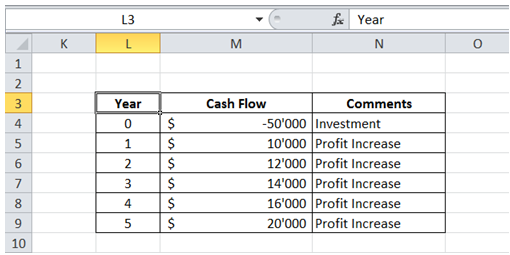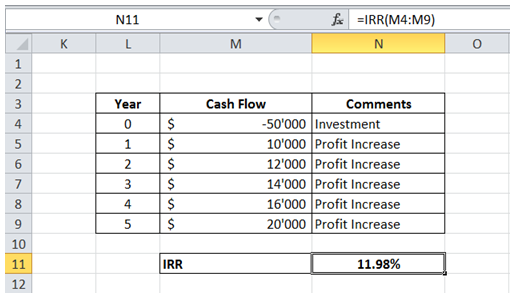## Cash flow how to calculated irr internal rate return in Excel

For example, I am considering making a \$50'000 investment that would increase my profits over the next 5 years as shown in Table below.To do it in Excel, here is the answer:

a) Enter the formula =IRR(M4:M9) as shown below. The formula returns the rate of Return for investment being considered.